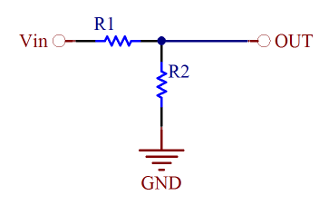# Voltage Divider Calculator

One of the most basic and common circuits is the two resistor voltage divider. The ratio of the resistors divides and input voltage down to a lower voltage. This calculator simplifies the task.Voltage Divider Circuit Schematic

 Inputs: Input Voltage: (V) Results: Output Voltage:(V) Ratio (R1/R2): Fill in one of the parameters below and the other two will be computed. R1 (KOhms) R2 (KOhms) R1+R2 (KOhms)

See our standard resistor calculator for a real world resistor value.

### Equations:

Vo= Vin*R2/(R1+R2)

R2/R1= Vo/(Vin-Vo)

[Plant Database], [Soil Moisture Sensor] [Water Level Sensor] [Soil Moisture Meter]